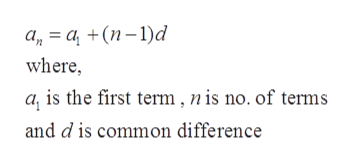# Find a formula for the problem below:7th term is 31; 20th term is 96

Question
27 views

Find a formula for the problem below:

7th term is 31; 20th term is 96

check_circle

star
star
star
star
star
1 Rating
Step 1

Given that

7th term is 31 and 20th term is 96

Step 2

To find the formula

Step 3

It is known that the nth term of an arithm...help_outlineImage Transcriptionclosea=a (n-1)d where a, is the first term,nis no. of terms and d is common difference fullscreen

### Want to see the full answer?

See Solution

#### Want to see this answer and more?

Solutions are written by subject experts who are available 24/7. Questions are typically answered within 1 hour.*

See Solution
*Response times may vary by subject and question.
Tagged in

### Other